# Test: Determinants (CBSE Level) - 2

## 25 Questions MCQ Test Mathematics (Maths) Class 12 | Test: Determinants (CBSE Level) - 2

Description
This mock test of Test: Determinants (CBSE Level) - 2 for JEE helps you for every JEE entrance exam. This contains 25 Multiple Choice Questions for JEE Test: Determinants (CBSE Level) - 2 (mcq) to study with solutions a complete question bank. The solved questions answers in this Test: Determinants (CBSE Level) - 2 quiz give you a good mix of easy questions and tough questions. JEE students definitely take this Test: Determinants (CBSE Level) - 2 exercise for a better result in the exam. You can find other Test: Determinants (CBSE Level) - 2 extra questions, long questions & short questions for JEE on EduRev as well by searching above.
QUESTION: 1

###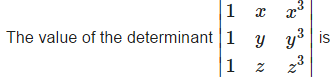Solution: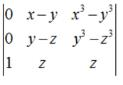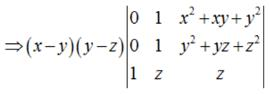⇒ (x-y)(y-z)[y2 + yz+z2 - x2 - xy - y2]

⇒ (x-y)(y-z) (z-x) (x+y+z)

QUESTION: 2

### If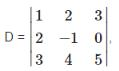, then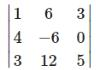is equal to

Solution: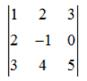=1(-5) -2(10) + 3(11)
=-5-20+33 = 8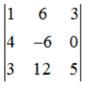=1(-30) - 6(20) + 3(66)

= -30-120+198= 48

D = 8 ⇒ 6D = 48

QUESTION: 3

###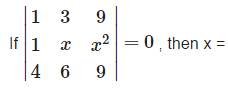Solution: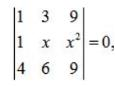Apply, R2 →R2 - R1,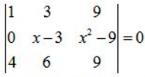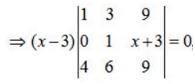Apply, R3 →R3 - R1,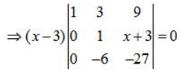⇒ (x-3) (6x -9)  = 0 ⇒x =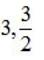QUESTION: 4

If the order of matrix A is m*p. And the order of B is p×n. Then the order of matrix AB is ?

Solution:
QUESTION: 5

If each element of a 3 × 3 matrix A is multiplied by 3 , then the determinant of the newly formed matrix is

Solution:
QUESTION: 6

One root of the equation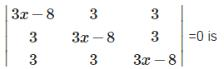Solution: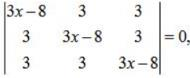Apply , R1→R1 +R2+R3,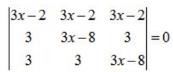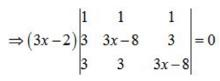⇒either (3x -2) = 0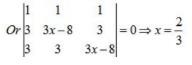QUESTION: 7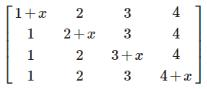Solution: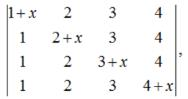Apply , C1 → C1+C2+C3+C4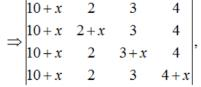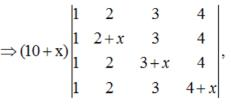Apply, R1 → R1 - R2,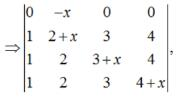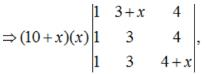Apply, R1→R1 - R2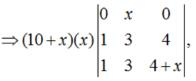⇒ (10+ x) x3

QUESTION: 8

The determinant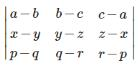is equal to

Solution: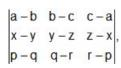Apply, C1 → C+ C2 + C3,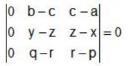QUESTION: 9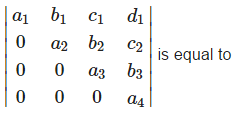Solution:

The determinant of a lower triangular (or an upper triangular matrix is equal to the product of the diagonal elements.

QUESTION: 10

If A is a non singular matrix of order 3 , then |adj(adjA)|

Solution: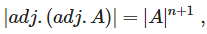where n is order of matrix. Here n = 3.

QUESTION: 11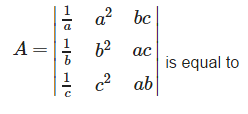Solution: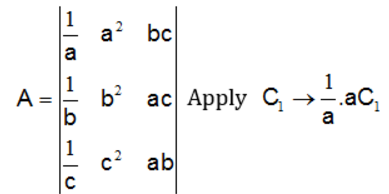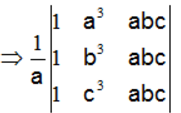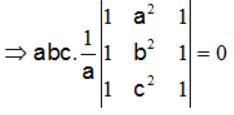QUESTION: 12

The only integral root of the equation det.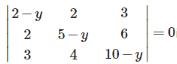is

Solution:

Clearly , y = 1 satisfies it. [C3 = 3C1]

QUESTION: 13

If 1/a+1/b+ 1/c = 0 , then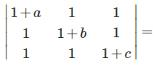Solution:

Since ,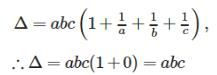QUESTION: 14

If A is a non singular matrix and A’ denotes the transpose of A , then

Solution:

Because , |A|=|A′|

QUESTION: 15

If the matrix AB = O , then

Solution:

If the matrix AB = O , then , marix A can be a non zero matrix as well as matrix B can be a non zero matrix. Which means det.A = 0 and det.B = 0.

QUESTION: 16

If A ,B andC be the three square matrices such that A = B + C , then Det A is equal to

Solution:

Because , |A| ≠ |B|+|C|

QUESTION: 17

Solution set of the equation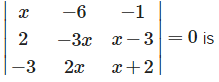Solution:

[x(-3x(x+2) - 2x(x-3)]+6[2(x+2)+3(x-3)]-1(4x-9x) = 0

⇒ - 5x3 + 35x - 30 = 0
⇒ (x-1)(x-2)(x+3) = 0 ⇒ x=1,2,-3

QUESTION: 18

A determinant is unaltered , if

Solution:

This is because of the elementary transformations of determinants . The value of determinant remains unaffected by applying elementary transformations.

QUESTION: 19

If A and B are square matrices of order 3 , such that Det.A = –1 , Det.B = 3 then the determinant of 3AB is equal to

Solution:

|3AB| = 27|A||B| = 27(-1)(3) = -81

QUESTION: 20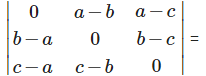Solution:

Because , the determinant of a skew symmetric matrix of odd order is always zero and of even order is a non zero perfect square.

QUESTION: 21

If 1 , ω,ω2 are cube roots of unity , then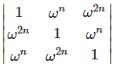has value

Solution:

write 1 as in R1 and take out  common from R1 , we get :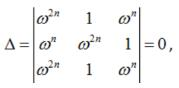because row 1 and row 3 are identical.

QUESTION: 22

Solution set of the equation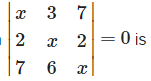Solution:

x(x2 - 12) - 3(2x - 14)+7(12 - 7x) = 0

⇒ x3 - 67x + 126 = 0

⇒(x-2)(x-7)(x+9) = 0 ⇒x = 2,7,-9

QUESTION: 23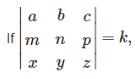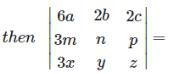Solution: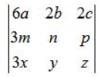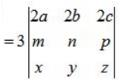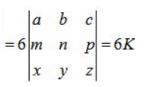QUESTION: 24

The system AX = B of n equations in n unknowns has infinitely many solutions if

Solution:

Explanation here if det. A = 0 , (adj A) B = O ⇒ The system AX = B of n equations in n unknowns may be consistent with infinitely many solutions or it may be inconsistent.

QUESTION: 25

If A is a square matrix such that A3 = I , then A−1 is equal to

Solution:

A3 = I ⇒  Pre - multiplying both sides by A−1,A−1, A3 = A−1 I ⇒ A2 = A−1